Next: Behavior of Density of Up: Statistical Mechanics Previous: Reversibility and Irreversibility

# Probability Calculations

The principle of equal a priori probabilities is fundamental to all of statistical mechanics, and allows a complete description of the properties of macroscopic systems in equilibrium. In principle, statistical mechanics calculations are very simple. Consider a system in equilibrium that is isolated, so that its total energy is known to have a constant value lying somewhere in the range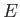to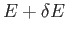. In order to make statistical predictions, we focus attention on an ensemble of such systems, all of which have their energy in this range. Let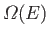be the total number of different states in the ensemble with energies in the specified range. Suppose that, among these states, there are a number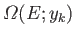for which some parameter,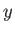, of the system assumes the discrete value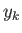. (This discussion can easily be generalized to deal with a parameter that can assume a continuous range of values.) The principle of equal a priori probabilities tells us that all of theaccessible states of the system are equally likely to occur in the ensemble. It follows that the probability,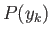, that the parameterof the system assumes the valueis simply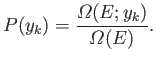(3.18)

Clearly, the mean value offor the system is given by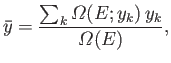(3.19)

where the sum is over all possible values thatcan assume. In the previous formula, it is tacitly assumed that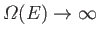, which is generally the case in thermodynamic systems.

It can be seen that, using the principle of equal a priori probabilities, all calculations in statistical mechanics reduce to counting states, subject to various constraints. In principle, this is a fairly straightforward task. In practice, problems arise if the constraints become too complicated. These problems can usually be overcome with a little mathematical ingenuity. Nevertheless, there is no doubt that this type of calculation is far easier than trying to solve the classical equations of motion (or Schrödinger's equation) directly for a many-particle system.Next: Behavior of Density of Up: Statistical Mechanics Previous: Reversibility and Irreversibility
Richard Fitzpatrick 2016-01-25